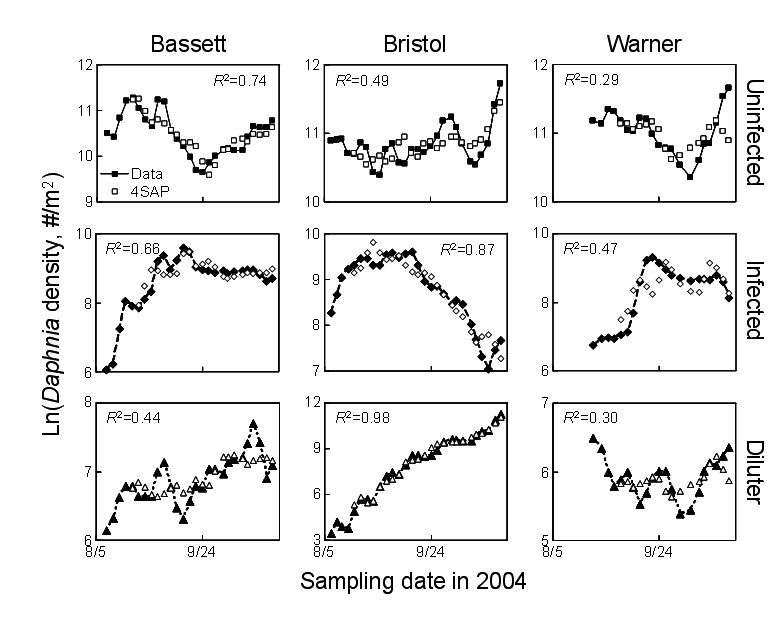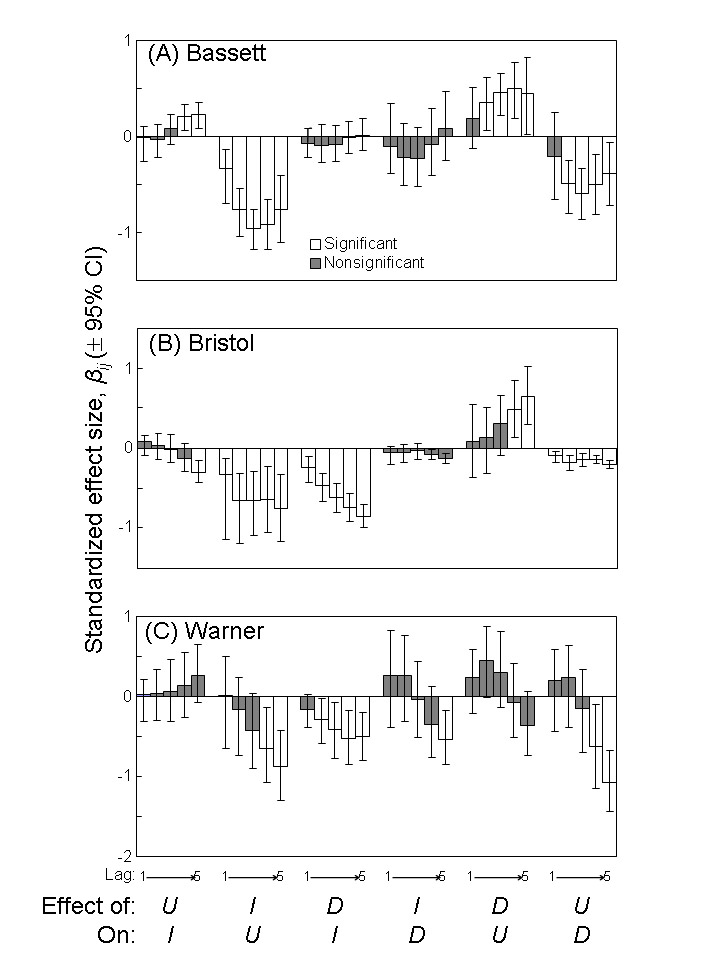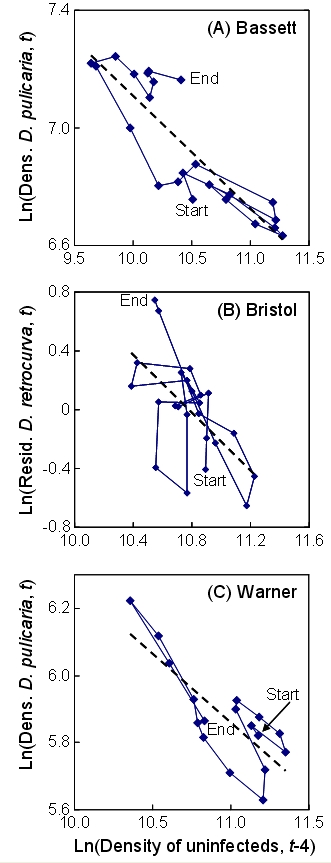### Spencer R. Hall, Claes R. Becker, Joseph L. Simonis, Meghan A. Duffy, Alan J. Tessier, and Carla E. Cáceres. 2009. Friendly competition: evidence for a dilution effect among competitors in a planktonic host–parasite system. Ecology 90:791–801.

Appendix A. More methods and results.

In this Appendix, we provide further description of methods and results stemming from fits of the multivariate autoregressive (MAR) time-series models.

More methods for the MAR modeling

To estimate the parameters of the multivariate autoregressive (MAR) models and confidence intervals around them, we followed the conditional least squares (CLS) approach outlined by Ives et al. (2003). The CLS method to estimate parameters is straightforward. For a given variable, we fit intercept and slope parameters using least squares. Given these parameter estimates, we looked for systematic patterns involving means, variances, and autocorrelation of errors (but not normality, since the bootstrapping procedure that we used does not assume it). Then, after fitting three equations (i.e., one like equation 1 for uninfected hosts and similar ones for infected hosts and diluters), we bootstrapped 95% confidence intervals (with 10,000 bootstraps). To create these intervals, we created bootstrapped time series by starting at an initial date (at τ -1), then iterating equation (2) from time τ until the last date of the series with triplets of residuals, E(t), sampled with replacement. This triplet-method is important because it maintains inherent, multivariate correlation structure among these residuals (Ives et al. 2003). Afterwards, we fit the model (Eqs. 2, 3) to each of the bootstrapped datasets using CLS, collected and sorted the bootstrapped parameter estimates, and choose the 2.5th and 97.5th percentiles from those distributions to determine 95% CIs. Finally, we fit these models twice to data smoothed with a three-point running average (with weights [0.25, 0.50, 0.25]). For plotting purposes, we used untransformed data (Fig. A1); then, for presentation of coefficients, we first standardized the data by dividing the time series for each variable by its standard deviation (Figs. 4, A2).

Additional results from estimation of the MAR models

In Fig. A1, we show fits of the MAR(4) model to actual time series data. We chose to present results from models estimated at a lag of four sampling visits (approximately 12 days). We chose a lag greater than one sampling visit for two reasons. First, a priori we expect to find that densities of hosts best related to each other at lags greater than one sampling visit, due to lags in diagnosis of infection (i.e., it takes over six days [two sampling visits] to see evidence of infection), but also due to delayed feedback between Daphnia competing for shared algal resources (which is indirect and could occur over several sampling visits). Second, lags of four (or even five) empirically captured the most signal from the data. Lags of one to three sampling visits conveyed less information (judged by significance of interaction coefficients; Fig. A2). For the most part, directionality of coefficients were consistent across lags (whether they were significantly different from zero or not). Unfortunately, we lack a good method to differentiate among MAR models fit at different lags. Therefore, at the present time, we can only offer these various results to the reader. Due to the (relative) shortness of the time series models, we did not fit lags higher than 5.FIG. A1. Time series data collected during intensive sampling of three epidemics in 2004. Data are ln-transformed areal densities of three types of hosts (uninfected and infected Daphnia dentifera or “diluters”, either D. pulicaria in Bassett and Warner or D. retrocurva in Bristol). Black symbols are data, open symbols are step ahead predictions set at a lag of four sampling visits (“4SAP”). Therefore, the differences between the open symbols and the closed ones capture the variation left unexplained by the models. The coefficient of determination (R2) values capture the variance explained by each MAR(4) model for each species/host class.FIG. A2. Analog of Fig. 4 but showing standardized effect sizes from the multivariate autoregressive models fit with lags (τ) of one to five sampling visits. The standardized coefficients (βij) related densities of a host class at sampling visits in the past to densities of another host classes in the present. Key to host classes: “U” = uninfected Daphnia dentifera; “I” = infected; “D” = diluter, either D. pulicaria (Bassett and Warner Lakes) or D. retrocurva (Bristol Lake). Error bars represent 95% confidence intervals (10,000 bootstraps); coefficients with 95% CIs that did not overlap zero were deemed “significant” (white), while those that did overlap zero were considered not significant (gray).FIG. A3. Illustrations of the negative relationships between density of uninfected hosts in the past (τ = 4) with density of “diluters” in the present in (A) Bassett, (B) Bristol, and (C) Warner Lakes during the intensively sampled 2004 epidemics. In (A) and (C), this relationship emerges clearly enough viewing the raw data. In (B) Bristol Lake, the negative relationship between uninfected hosts and diluters appears after accounting for strong positive autocorrelation between density of D. retrocurva in the past (τ = 4) with D. retrocurva in the present. Hence, for this lake, we use residuals to illustrate the pattern. The regressions lines are intended to show directionality of these relationships; in the MAR(4) models, the multiple regression coefficients account for autocorrelation between past and present density of diluters and relationships between past uninfected and present diluter density. All densities are on a natural log scale.

LITERATURE CITED

Ives, A. R., B. Dennis, K. L. Cottingham, and S. R. Carpenter. 2003. Estimating community stability and ecological interactions from time-series data. Ecological Monographs 73:301–330.

[Back to E090-054]